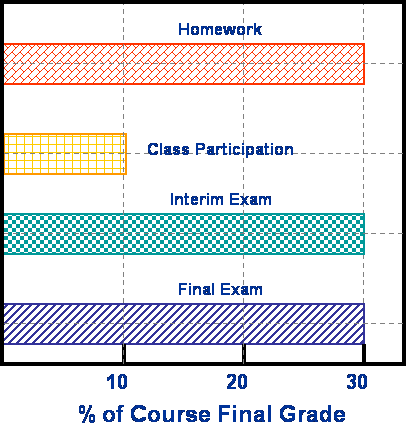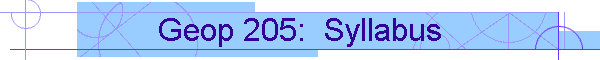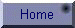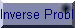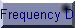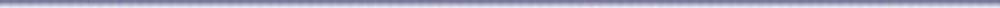GEOP. 205
COMPUTATIONAL GEOPHYSICS

Bulletin Data:

 Geop. 205: Computational Geophysics      (3 0 3) Topics covered include: Fourier transform; partial differential equations of geophysics; linear operators; convolution; correlation techniques; digital filters; the FFT algorithm; analytic continuation; probability distributions; trend surface analysis; with emphasis on computer applications of these tools to geophysical data; MATLAB would be used to illustrate the techniques numerically. Prerequisites: MATH 201, ICS 103 and GEOP 202

Instructor:    Dr. A. Abokhodair

Textbook:

A.R. Camina and G.J. Janacek; Mathematics for Seismic Data Processing and Interpretation.

References:

1. Reading material on my web site

2. Riley; Mathematical Methods for the Physical Sciences2.

3. Wonnacott and Wonnacott; Introductory Statistics

4. Bloomfield; Fourier Analysis of Time Series: An Introduction

5. Brigham; The Fast Fourier Transform

6. Menke; Geophysical Data Analysis: Discrete Inverse Theory

Instructional Goals:

Students are expected to: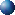Understand the two main objectives of geophysical data processing-namely, enhancement and modeling and parameter estimation.Gain experience in computer usage to solve numerical problems in geophysics.Develop greater familiarity with certain mathematical tools and constructs [Vectors, Matrices, Transforms] as used in geophysical computation.Understand the basic principles of geophysical data inversion, parameter estimation, assessment of solution goodness.Understand the basic principles of filtering and its applications for data enhancement.

Essential Prerequisites:

In addition to the course prerequisites stated in the Bulletin, students are expected to have completed their general mathematics and physics course requirements.

Vector Analysis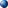Vector algebra, calculus and operators.Some important concepts and theorems: the gradient, the divergence, and the curl; Gauss, Green, and Stockes’ theorems.Examples of geophysical applications of vectors: Gravity and Magnetic fields and field equations.SVD; Paleomagnetic data analysis.
1

Matrix ComputationBasic concepts from linear algebra: vector space, bases vectors, and linear independence.Matrix algebra: addition, multiplication, and inversion of matrices.Matrix rank and linear dependence: singular matrices and some special types of square matrices.Matrix functions: their integration and differentiation.
2

Elements of StatisticsBasic Concepts: classification of data, histograms, and frequency distribution; mean, variance, standard deviation, and moments.Elements of probability theory and sampling: sample space, sample point and events; conditional probability and independence of events.Discrete and continuous random variables: important probability distribution of random variables.
1

Elements of Inverse TheoryBasic concepts: observational data, random error, model parameters, and measures of vector length.Classification and Formulation of inverse problems: The implicit and the explicit linear linear forms. Overdetermined, Evendetermined, and Underdetermined linear inverse problems.Solutions of LIP: prediction error, vector norms, and the solution of the Overdetermined problem; priori information, constraints, Lagrange multipliers, and the solution of the Underdetermied problem.Generalized inverse: measures of goodness of resolution and covariance; the data resolution, the model resolution, and the unit covariance matrices.Description of some Linear Inverse Problems [LIP] in geophysics with examples from gravity, magnetic and seismic exploration methods.Generalized inverse: measures of goodness of resolution and covariance: the data resolution, the model resolution, and the unit covariance matrices. Description of some Linear Inverse Problems [LIP] in geophysics with examples from gravity, magnetic and seismic exploration methods.
4

Fourier TransformFourier Analysis and Synthesis: The Fourier series and properties; expansion of functions; discrete form; computation of coefficients by inversion.Applications: Continuation of potential fields.Fourier transform and inverse transform: transform pairs of special functions, even, odd and mixed functions; useful properties of the Fourier transform.The Discrete (DFT) and Fast Fourier (FFT) Transforms; some algorithms for their computation.
2

Filtering FundamentalsBasics of Signal analysis: amplitude, period, wavelength, cycle, spatial, temporal, and angular frequencies.Sampling Theorem: sampling rate and aliasing.Basic concepts of linear systems: convolution, and digital filtering; Low Pass, High Pass, and Band Pass filters.Geophysical Examples: continuation and derivatives of potential fields, data smoothing, signal separation.Spectral Analysis: autocorrelation, crosscorrelation and power spectrum, windowing, simple methods of spectral estimation.Power spectra and the interpretation of magnetic anomalies.
4
 Computer Usage:  Heavy; Using MATLAB Laboratory:    Computer Lab, computational experiments. Project: One Major Project Grading: See figure to the right